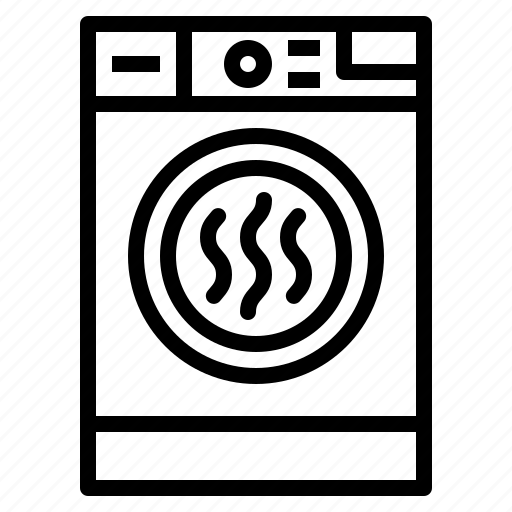## Energy Usage Cost Calculators

Use the below tools to calculate energy usage of your typical house-hold appliances and the associated costs. These are great to give you an idea of everyday running costs within your home. Just select an appliance and enter the required information.## Desktop PC- How much does it cost to use?

Note; Based on a typical desktop PC rated at 100 Watts and each monitor rated at 50 Watts in use## Dishwasher- How much does it cost to use?

Note; power consumption based on typical units. Actual power used will vary slightly depending on individual unit settings.## Fridge Freezer- How much does it cost to use?

Note; Based on a typical fridge freezer unit consuming 300 kWh per annum## Hairdryer- How much does it cost to use?

Note; Based on a typical hairdryer unit rated at 2 kW## Kettle- How much does it cost to use?

Note; Based on average mug size (330ml). Kettles will have slightly different power ratings but all will take roughly the same amount of energy to boil water, it is the amount of time it takes that will vary.## Laptop- How much does it cost to use?

Note; Based on a typical laptop rated at 50 Watts in use## Lighting- How much does it cost to use?

Note; to assess the cost of lighting a room per hour, select the output of each luminaire/bulb and the number of them.## Tablet- How much does it cost to use?

Note; Based on a typical tablet rated at 5 Watts in use## Television- How much does it cost to use?

Note; most modern TVs use approximately 0.5 Watts of power in standby mode. This is equivalent to 0.012 kWh per day or around 1 penny every 4 days (depending on energy tariff).## Tumble Dryer- How much does it cost to use?

Note; power consumption based on typical units. Heat pump style units tend to operate at lower temperatures for longer drying times.## Vacuum cleaner- How much does it cost to use?

Note; power consumption based on an average vacuum cleaner. Figures work for both wired and battery powered units.## Washing Machine- How much does it cost to use?

Note; power consumption based on typical units. Actual power used will vary slightly depending on individual unit settings, wash temperature etc.